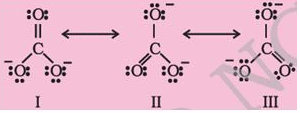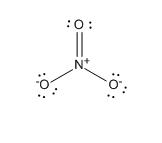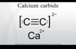Courses

# Chemical Bonding And Molecular Structure Practice Test (1)

## 25 Questions MCQ Test Additional Question Bank | Chemical Bonding And Molecular Structure Practice Test (1)

Description
This mock test of Chemical Bonding And Molecular Structure Practice Test (1) for Class 9 helps you for every Class 9 entrance exam. This contains 25 Multiple Choice Questions for Class 9 Chemical Bonding And Molecular Structure Practice Test (1) (mcq) to study with solutions a complete question bank. The solved questions answers in this Chemical Bonding And Molecular Structure Practice Test (1) quiz give you a good mix of easy questions and tough questions. Class 9 students definitely take this Chemical Bonding And Molecular Structure Practice Test (1) exercise for a better result in the exam. You can find other Chemical Bonding And Molecular Structure Practice Test (1) extra questions, long questions & short questions for Class 9 on EduRev as well by searching above.
QUESTION: 1

### Octet of electrons, represents a particularly stable electronic arrangement.. Atoms achieve the stable octet when they are linked by chemical bonds. This rule is associated with one of the following theories

Solution:

Lewis theory states that elements in order to get stabalised completes their octet.

QUESTION: 2

### Structures of CO2−3CO32−. ion given below explains the phenomenon of in terms ofSolution:

Resonance is the phenomenon when a molecule can be represented by 1 or more structure differing in the position of bonds and charge.

QUESTION: 3

### The maximum number of hydrogen bonds that a molecule of water can have is

Solution:

a molecule of water can form max of 4 Hydrogen bonds.

QUESTION: 4

For a stable molecule the value of bond order must be

Solution:

stable molecule have positive value of bond order.

QUESTION: 5

Polarity in a molecule and hence the dipole moment depends primarily on electronegativity of the constituent atoms and shape of a molecule. Which of the following has the highest dipole moment?

Solution:

Because of presence of lp on head of O atom in H2O therefore it has max dipole moment.

QUESTION: 6

Inertness of noble gas was observed to be due to their electronic configurations: Choose the most appropriate

Solution:

Noble gases have fully filled octet in their valence shell.​

QUESTION: 7

The product of the magnitude of the charge and the distance between the centres of positive and negative charge.

Solution:

µ=q x r

QUESTION: 8

During the formation of a chemical bond

Solution:

chemical bond formation leads to stability in system hence energy decreases.

QUESTION: 9

In acetylene molecule, between the carbon atoms there are

Solution:

acetylene is C2H2 C atoms are bound with triple bond i.e. 1 sigma and 2 pie bonds.

QUESTION: 10

The types of hybrid orbitals of nitrogen in No+2, No−3 and NH+4 respectively are expected to be

Solution:

hybridization of N in No2+, No3 and NH4+ respectively are sp, sp3and sp2

QUESTION: 11

Stable outer octet of electrons is achieved in chlorine atom during the formation of NaCl by:

Solution:

Na lose its one electron forming Na+ while Cl gains the electron formimg Cl- .​

QUESTION: 12

the valence shell electron pair repulsion (vsepr) theory helps in the

Solution:

VSPER helps in predicting the geometrical shapes of molecules.

QUESTION: 13

Which of the following has maximum number of lone pairs associated with Xe?

Solution:

XeF2 will have 2 bp while 3 lps.

QUESTION: 14

In PO3−4  ion the formal charge on the oxygen atom of P–O bond is

Solution:

Formal charge on the oxygen atom of P–O bond is – 1

QUESTION: 15

Isostructural species are those which have the same shape and hybridisation. Among the given species identify the isostructural pairs.

Solution:

BF4−andNH+4 both have sp3 hybridisation.

QUESTION: 16

The bond formed, as a result of the electrostatic attraction between the positive and negative ions was termed as

Solution:

electrovalent or ionic bond is nothing but electrostatic force of attraction between positive cation and negative anion.

QUESTION: 17

In the formation of hydrogen molecule, overlapping of atomic orbitals which results in the pairing of electrons. These are:

Solution:

overlapping of atomic orbitals having electrons of opposite spin take palce in the formation of molecule.

QUESTION: 18

The structure of IF7is

Solution:

Steric no of IF7 is 7 so geometry and structure is pentagonal bipyramidal.

QUESTION: 19

In which of the following substances will hydrogen bond be strongest?

Solution:

Out of all H2O will form strongest hydrogen bonding due to high electronegativity of O.

QUESTION: 20

In NO3 ion, the number of bond pairs and lone pairs of electrons on nitrogen atom are

Solution:

In NO3 ion number of bond pair and lone pair of electrons on nitrogen atom respectively are :  4,0.QUESTION: 21

The number of dots around the Lewis symbols for the elements represent

Solution:

dots reprents the valence electrons.

QUESTION: 22

In NO3 ion, the number of bond pairs and lone pairs of electrons on nitrogen atom are

Solution:

NO3 have 3 bps and 0 lps.

QUESTION: 23

The number of types of bonds between two carbon atoms in calcium carbide is

Solution:CaC2 have one sigma and 2 pie bonds.

QUESTION: 24

When a gas phase atom in its ground state gains an electron. This is called

Solution:

electron gain enthalpy is the energy released when an electron is gained by isolated gaseous atom to form isolated gaseous anion.

QUESTION: 25

Amongst the following elements whose electronic configurations are given below, the one having the highest ionisation enthalpy is

Solution:

A and D both have stable half filled configuration but in A the atomic radii is samller than D so IE of A is highest.